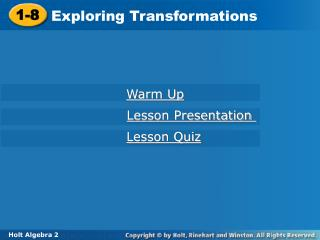DownloadDownload Presentation1-8

# 1-8

Download Presentation## 1-8

- - - - - - - - - - - - - - - - - - - - - - - - - - - E N D - - - - - - - - - - - - - - - - - - - - - - - - - - -
##### Presentation Transcript

1. 1-8 Exploring Transformations Warm Up Lesson Presentation Lesson Quiz Holt Algebra 2

2. Objectives Apply transformations to points and sets of points. Interpret transformations of real-world data.

3. Vocabulary transformation translation reflection stretch compression

4. A transformation is a change in the position, size, or shape of a figure. A translation, or slide, is a transformation that moves each point in a figure the same distance in the same direction.

5. Example 1A: Translating Points Perform the given translation on the point (–3, 4). Give the coordinates of the translated point. 5 units right 5 units right  (-3, 4) (2, 4) Translating (–3, 4) 5 units right results in the point (2, 4).

6. 2 units 3 units  (–5, 2) Example 1B: Translating Points Perform the given translation on the point (–3, 4). Give the coordinates of the translated point. 2 units left and 2 units down (–3, 4)  Translating (–3, 4) 2 units left and 2 units down results in the point (–5, 2).

7. Notice that when you translate left or right, the x-coordinate changes, and when you translate up or down, the y-coordinate changes.

8. A reflection is a transformation that flips a figure across a line called the line of reflection. Each reflected point is the same distance from the line of reflection, but on the opposite side of the line.

9. You can transform a function by transforming its ordered pairs. When a function is translated or reflected, the original graph and the graph of the transformation are congruent because the size and shape of the graphs are the same.

10. Example 2A: Translating and Reflecting Functions Use a table to perform each transformation of y=f(x). Use the same coordinate plane as the original function. translation 2 units up

11. Example 2A Continued translation 2 units up Identify important points from the graph and make a table. Add 2 to each y-coordinate. The entire graph shifts 2 units up.

12. Example 2B: Translating and Reflecting Functions reflection across x-axis Identify important points from the graph and make a table. Multiply each y-coordinate by –1. The entire graph flips across the x-axis.

13. Check It Out! Example 2a Use a table to perform the transformation of y = f(x). Use the same coordinate plane as the original function. translation 3 units right Add 3 to each x-coordinate. The entire graph shifts 3 units right.

14. Check It Out! Example 2b Use a table to perform the transformation of y = f(x). Use the same coordinate plane as the original function. reflection across x-axis f Multiply each y-coordinate by –1. The entire graph flips across the x-axis.

15. Imagine grasping two points on the graph of a function that lie on opposite sides of the y-axis. If you pull the points away from the y-axis, you would create a horizontal stretch of the graph. If you push the points towards the y-axis, you would create a horizontal compression.

16. Stretches and compressions are not congruent to the original graph. Stretches and Compressions

17. Example 3: Stretching and Compressing Functions Use a table to perform a horizontal stretch of the function y = f(x)by a factor of 3. Graph the function and the transformation on the same coordinate plane. Identify important points from the graph and make a table. Multiply each x-coordinate by 3.

18. Check It Out! Example 3 Use a table to perform a vertical stretch of y = f(x) by a factor of 2. Graph the transformed function on the same coordinate plane as the original figure. Identify important points from the graph and make a table. Multiply each y-coordinate by 2.

19. Example 4: Business Application The graph shows the cost of painting based on the number of cans of paint used. Sketch a graph to represent the cost of a can of paint doubling, and identify the transformation of the original graph that it represents. If the cost of painting is based on the number of cans of paint used and the cost of a can of paint doubles, the cost of painting also doubles. This represents a vertical stretch by a factor of 2.

20. Check It Out! Example 4 Recording studio fees are usually based on an hourly rate, but the rate can be modified due to various options. The graph shows a basic hourly studio rate.

21. If the price is discounted by of the hourly rate, the value of each y-coordinate would be multiplied by . Check It Out! Example 4 Continued What if…? Suppose that a discounted rate is of the original rate. Sketch a graph to represent the situation and identify the transformation of the original graph that it represents.